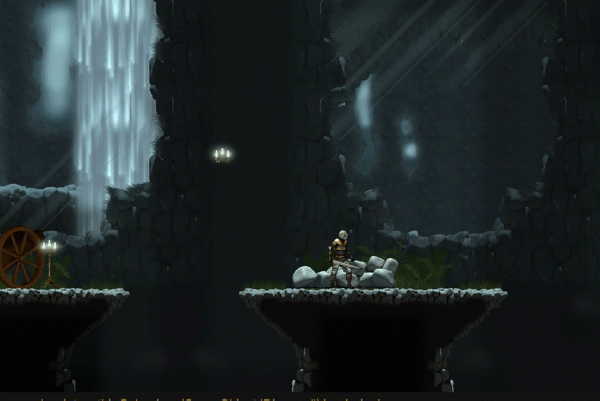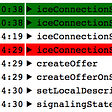# Build Array from Permutation — Day 103(Python)

Today, we will look into another easy problem.

1920. Build Array from Permutation

Given a zero-based permutation `nums` (0-indexed), build an array `ans` of the same length where `ans[i] = nums[nums[i]]` for each `0 <= i < nums.length` and return it.

A zero-based permutation `nums` is an array of distinct integers from `0` to `nums.length - 1` (inclusive).

Example 1:

`Input: nums = [0,2,1,5,3,4]Output: [0,1,2,4,5,3]Explanation: The array ans is built as follows: ans = [nums[nums], nums[nums], nums[nums], nums[nums], nums[nums], nums[nums]]    = [nums, nums, nums, nums, nums, nums]    = [0,1,2,4,5,3]`

Example 2:

`Input: nums = [5,0,1,2,3,4]Output: [4,5,0,1,2,3]Explanation: The array ans is built as follows:ans = [nums[nums], nums[nums], nums[nums], nums[nums], nums[nums], nums[nums]]    = [nums, nums, nums, nums, nums, nums]    = [4,5,0,1,2,3]`

Constraints:

• `1 <= nums.length <= 1000`
• `0 <= nums[i] < nums.length`
• The elements in `nums` are distinct.

Follow-up: Can you solve it without using an extra space (i.e., `O(1)` memory)?

We are given a zero-based permutation, which means in our array we have numbers from 0 to length(nums)-1. We need to return an array whose elements are positioned as nums[nums[i]].

`class Solution:    def buildArray(self, nums: List[int]) -> List[int]:        output = []        for n in nums:            output.append(nums[n])        return output`

Complexity

Time Complexity

Since we are just traversing through the array once, the time complexity is O(N).

Space Complexity

We are using extra space to store our result, hence space complexity is O(N).

Let us try to solve the follow-up question.

`class Solution:    def buildArray(self, nums: List[int]) -> List[int]:        return( nums[nums[i]] for i in range(len(nums)))`

Here we are running for-loop inside the return statement itself.

Time Complexity

Since we are just traversing through the array once, the time complexity is O(N).

Space Complexity

We are not using any extra space, and hence the space complexity will be O(1).

--

--

--

## More from Annamariya Tharayil

Software Engineer. Find me @ www.linkedin.com/in/annamariya-jt

Love podcasts or audiobooks? Learn on the go with our new app.

## Creating automatic reports with Jira and PowerBI## Python keywords and identifiers..## 2D Mobile Game: Damage Interface## Getting started with VS CODE remote development## Gone in 1100 seconds — The weirdest bug I have ever met## Show Your DeviantArt in Augmented Reality!## Introducing tackr: Thumbtack’s Customized R Package## Annamariya Tharayil

Software Engineer. Find me @ www.linkedin.com/in/annamariya-jt

## 12 Best C Programming projects for Beginners## Is your code too complicated?## Advent Of Code 2021 — Smoke Basin — Puzzle 9## Coding improves problem solving